Browse by Topic
Related Topics

# Happy Vampire Day

I recently was asked about Fibonacci Day. I think I replied “What is Fibonacci Day?” Then the person explained it. November 23 is 11/23. Or 1, 1, 2, 3—the start of the Fibonacci sequence.

Other yearly math-related days I found were Pi Day (3/14), Foursquare Day (4/16), Pi Approximation Day (22/7, in European format), Opposite Day (12/21), and Mole Day (6:02 10/23).

A lot of these seem a bit arbitrary. I thought I might be able to do better, so here’s what I came up with for the month of September.

September 2010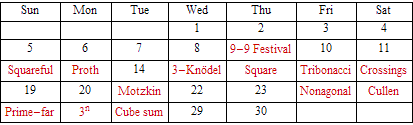The date 9/16 I have labeled as “Square”, along with a link to an explanatory page, since both 9 and 16 are consecutive square numbers. Both 9 and 25 are of the form 2n n+1, giving Cullen numbers the date 9/25.

October has a rather special day—10/05/2010 is a vampire date, since 10052010 = 5001 × 2010. The next two 8-digit vampire dates are 10/05/2064 and 10/19/2248. As a puzzle, try to figure out how to rearrange their digits into two 4-digit numbers, which have a product of the original number.

October 2010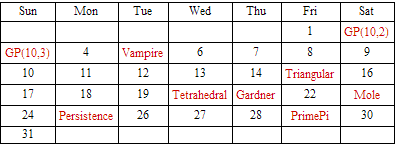October also has 10/2 and 10/3, which are generalized Petersen notations for the dodecahedral and Desargues graphs. Prime = 29, giving 10/29. Also look forward to 10/10/10 this month.

November and December also have interesting days, from a mathematical perspective. For example, the harmonic numbers sum up fractions 1/1, 1/2, 1/3, and so on, giving sums 3/2, 11/6, 25/12, and so on. I used the numerators for 11/25. These same numbers measure the possible overhang for a stack of books and the odds that you’ll fill a complete collection of something.

November 2010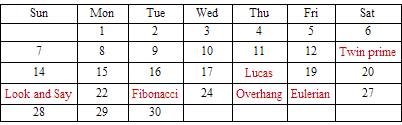December 2010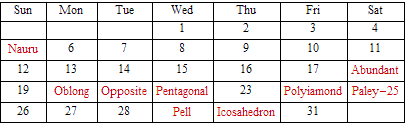The date 11/21 is a look and say day (one one, two ones). Ironically, next year is 2011, part of the self-descriptive sequence 0, 10, 2011, which describes everything previous (zero; one zero; two zeros, one one). Another day to look forward to is 12/11/10.

Here’s a picture for Paley-25 and its graph complement. For both graphs, each number connects to 12 of the 25 others. Every possible pair of numbers is on exactly one of the lines, which wrap around. For example, find both 12 and 25 on the bottom black line in the second picture. Four and 17 are on the red line in the first picture.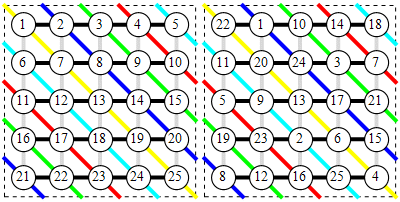All in all, there’s no shortage of additional mathematical days we could celebrate. Good ×, c’mon!